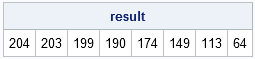Recently Charlie Huang showed how to use the SAS/IML language to compute an exponentially weighted moving average of some financial data. In the commentary to his analysis, he said:

I found that if a matrix or a vector is declared with specified size before the computation step, the program’s efficiency would be significantly improved. It may suggest that declaring matrices explicitly could be a good habit for SAS/IML programming.

Charlie has stated a general rule: in matrix/vector languages such as SAS/IML, MATLAB, or R, you should allocate space for a matrix outside of a loop, rather than using concatenation to grow the matrix inside of a loop. I cover this in Chapter 2 (p. 42-43) of my book, Statistical Programming with SAS/IML Software. You can download Chapter 2 at no cost from the book's Web site.

### Do Not Grow Arrays Dynamically

This general rule is relevant when you compute values of a matrix one element at a time. That is, you are using np separate computations to fill all elements of an n x p matrix.

Suppose that you want to compute a vector that contains the results of several similar computations. Naturally, you write a DO loop and compute each value within the loop. For example, the following SAS/IML statements define a SAS/IML module and call it eight times within a DO loop:

```proc iml; start Func(x); /** compute any quantity **/ return (ssq(x)); /** sums of squares **/ finish;   /** NOT EFFICIENT **/ /** grow the result array dynamically **/ do i = 1 to 8; x = i:8; result = result || Func(x); end; print result;```The program is not efficient because it uses the horizontal concatenation operator (||) to grow the result vector dynamically within the DO loop. After the ith iteration, the vector contains i elements, but during the ith iteration, the previous i – 1 elements are needlessly copied from the old array to the new (larger) array. If the DO loop iterates n times, then

1. the first element is copied n times
2. the second element is copied n – 1 times
3. the third element is copied n – 2 times, and so forth

In summary, when you grow an array dynamically within a loop, there are n allocations and n (n – 1) / 2 elements are needlessly copied.

### A Better Approach: Pre-Allocate the Array

When you know the ultimate size of an array, it is best to allocate the array prior to the loop. You can then assign values to the elements of the array inside the loop, as shown in the following statements:

```/** EFFICIENT: Pre-allocate result array **/ result = j(1, 8); do i = 1 to 8; x = i:8; result[i] = Func(x); end;```

This computation does not contain any needless allocations, nor any unnecessary copying of elements that were previously computed.

This technique is essential for efficient sampling in the SAS/IML language: when you want random values from a specified distribution (such as the normal distribution), you should pre-allocate a matrix and then call the RANDGEN subroutine once in order to fill the matrix with random numbers.

ShareDistinguished Researcher in Computational Statistics

Rick Wicklin, PhD, is a distinguished researcher in computational statistics at SAS and is a principal developer of SAS/IML software. His areas of expertise include computational statistics, simulation, statistical graphics, and modern methods in statistical data analysis. Rick is author of the books Statistical Programming with SAS/IML Software and Simulating Data with SAS.

1.Peter Borysov on

Hello Rick!

Thank you for your blog! I know that it is very difficult to come up with interesting ideas on a schedule:) Even though I am not using IML currently, I still can find a lot of very useful things here.

I am using Matlab, and I also find that if you have two nested loops, then it runs much faster if you set the inside loop to go through the rows and the outside loop to go through the columns, especially for big matrices. I think it has to do with the way the matrices are stored in the memory. How is it done in IML?

Peter

2.Rick Wicklin on

Yes, MATLAB and R both store their matrices column-wise. In contrast, SAS/IML software stores matrices row-wise. I haven't done the computation, but I'd wager that for large matrices it is more efficient to access the matrix in a way that is compatible with its storage structure.